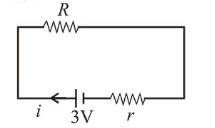# A battery of 3.0 VQuestion:

A battery of $3.0 \mathrm{~V}$ is connected to a resistor dissipating $0.5$ W of power. If the termial voltage of the battery is

$2.5 \mathrm{~V}$, the power dissipated within the internal resistance is:

1. (1) $0.50 \mathrm{~W}$

2. (2) $0.072 \mathrm{~W}$

3. (3) $0.10 \mathrm{~W}$

4. (4) $0.125 \mathrm{~W}$

Correct Option: , 3

Solution:

(3) When resistor is connected power dissipated,

$P_{R}=0.5 \mathrm{~W}$

Emf of battery, $E=3 \mathrm{~V}$ Terminal voltage, $V=2.5 \mathrm{~V}$$P_{R}=i^{2} R=0.5 \mathrm{~W}$

Also, $V=E-i r \Rightarrow 2.5=3-i r \Rightarrow i r=0.5$

Power dissipated across $' r^{\prime}: P_{r}=i^{2} r$

Now $i R=2.5$ and $i r=0.5$

$\therefore \frac{R}{r}=5$

Now $\frac{P_{R}}{P_{r}}=\frac{i^{2} R}{i^{2} r} \Rightarrow \frac{P_{R}}{P_{r}}=\frac{R}{r} \Rightarrow \frac{P_{R}}{P_{r}}=5$

$\Rightarrow P_{r}=\frac{P_{R}}{5}=\frac{0.50}{5}$

$\therefore P_{r}=0.10 \mathrm{~W}$